Get instant live expert help with Excel or Google Sheets“My Excelchat expert helped me in less than 20 minutes, saving me what would have been 5 hours of work!”

#### Post your problem and you’ll get Expert help in seconds.

Your message must be at least 40 characters
Our professional Expert are available now. Your privacy is guaranteed.

# How Do You Do Conditional Formatting with 2 Conditions?

The conditional formatting is used to create visual differentiation in a large set of data, in some standard format. Normally, the data can be visually differentiated using one or more rules, however, in this article, we will discuss how to apply conditional formatting with 2 conditions.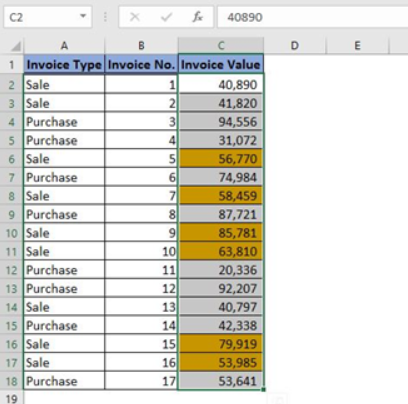Figure 1 – Final result

## 1 Column, 2 Rules, 2 Conditions

Let’s assume that we have a set of different Sales Invoice data and we want to segregate invoices using conditional formatting which are either below or above \$50,000. In order to achieve this, we need to follow the below steps:

• Select the data range containing the invoice values, click on “Conditional Formatting” available on “Home” tab.
• Choose “New Rule” from the drop-down menu.
• Choose the rule type “Format only cells that contain”. In the “Format only cells with” section, select “Cell Value” in the first drop-down box and “greater than” from the second drop down. Write 50000 in the value field.
• Click “Format” to display the Format Cells dialog box, choose the format as per your liking. (Figure 2)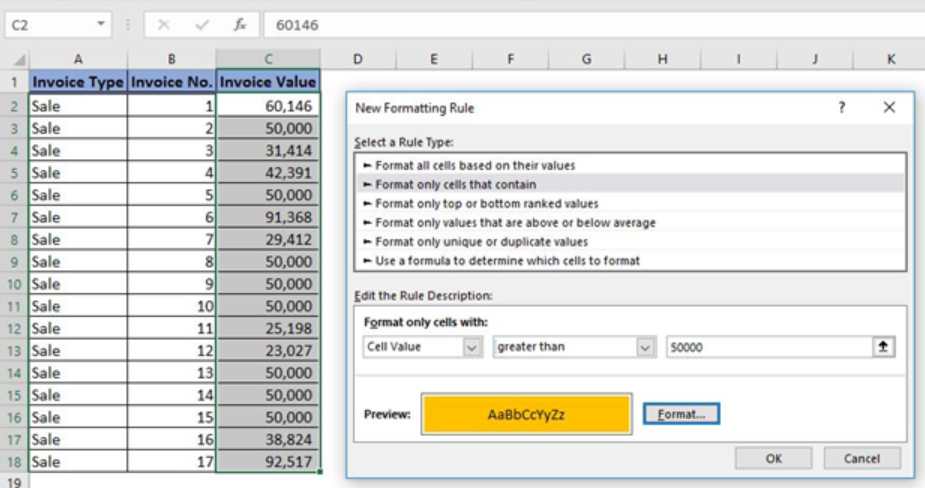Figure 2 – Selection and application of the first condition

• Click “OK” for the formatting to come into effect.
• After completing the above steps, the invoices having a value greater the \$50,000 will be highlighted. (Figure 3)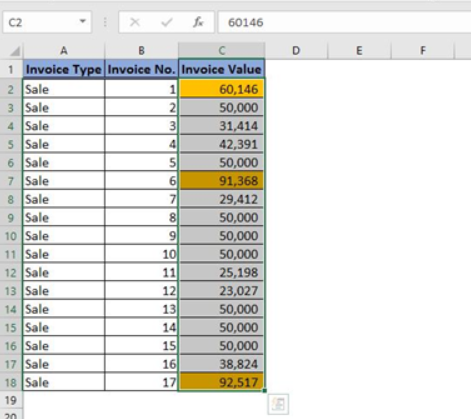Figure 3 – Result after application of the first condition

• We have applied the first condition, now, we will move on to the application of the second condition.
• The initial steps involved are exactly the same. We will again select the data range containing the invoice values then go to “Home>Conditional Formatting>New rule”
• Choose the rule type “Format only cells that contain”. In the “Format only cells with” section, select “Cell Value” in the first drop-down box and “less than” from the second drop down. Write 50000 in the value field.
• Click “Format” to display the Format Cells dialog box, choose the format as per your liking. (Figure 4)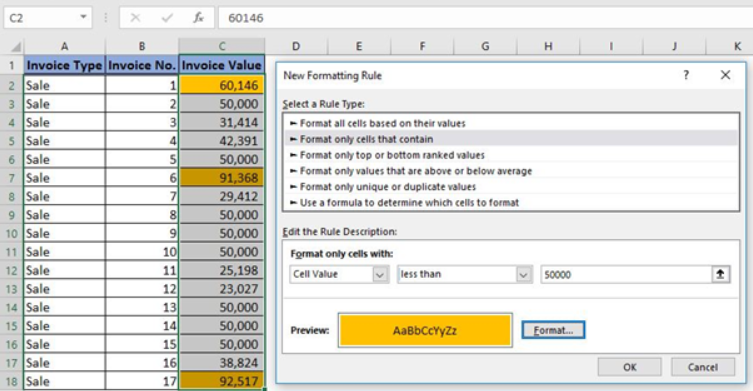Figure 4 – Selection and application of the second condition

• Click “OK” for the formatting to come into effect.
• The final result will be the highlighted invoice values that are either greater or lower than \$50,000. (Figure 5)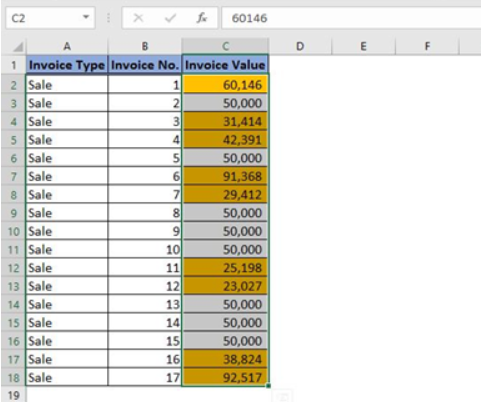Figure 5 – Final Result after application of 2 Conditions

• We can also view the rules that we have applied on our dataset by going to “Home>Conditional Formatting>Manage Rules”. (Figure 6)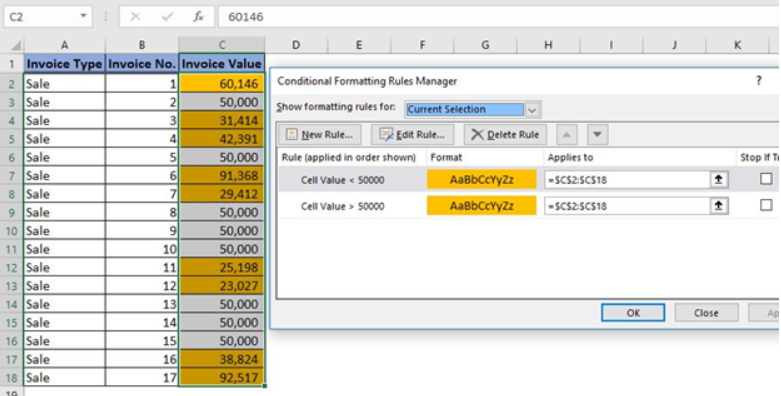Figure 6 – Rules showing 2 conditions applied to the same dataset

## 1 Column, 1 Rule, 2 Conditions

The second way through which we can apply two conditions on the same dataset is by using a formula. In this technique, the steps involved are as follows:

• Select the data range containing the invoice values.
• We will reach to the conditional formatting dialog box in the usual way i.e. “Home>Conditional Formatting>New rule”.
• Now instead of selecting rule type “Format only cells that contain”, we will select “Use a formula to determine which cells to format”.
• The formula that we will apply, to select our invoices that have a value of greater or lower than \$50,000, is `=OR(C2>50000,C2<50000)`
• Click “Format” to display the Format Cells dialog box, choose the format as per your liking. (Figure 7)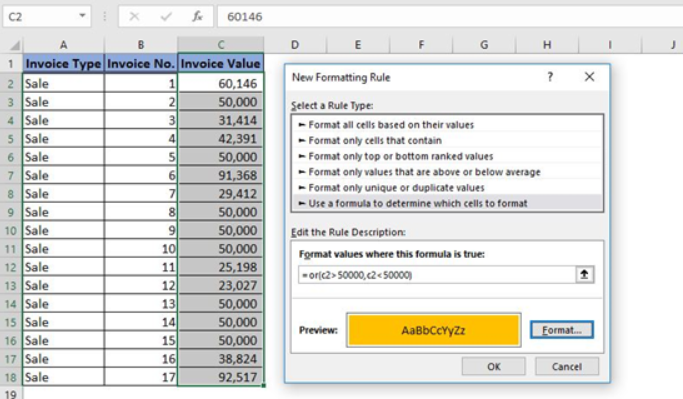Figure 7 – Application of formula to cater 2 conditions in 1 rule

In the above formula we have used “OR” operator to test two conditions to identify invoices having value either greater or lower than \$50,000. After application of formatting rules click “OK”. We will see the same results which we saw in the first technique. (Figure 8)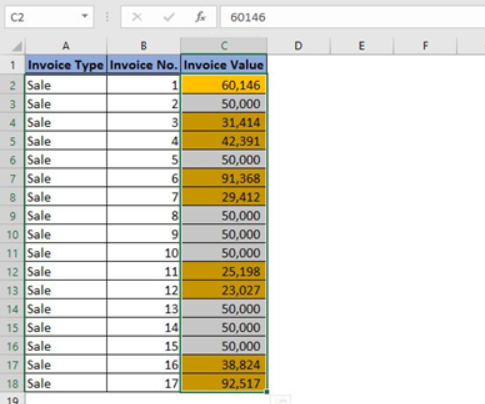Figure 8 – Result after application of conditional formatting using a formula

In the above scenario, the “Manage Rules” dialog box will show one rule applied to the dataset with two conditions. (Figure 9)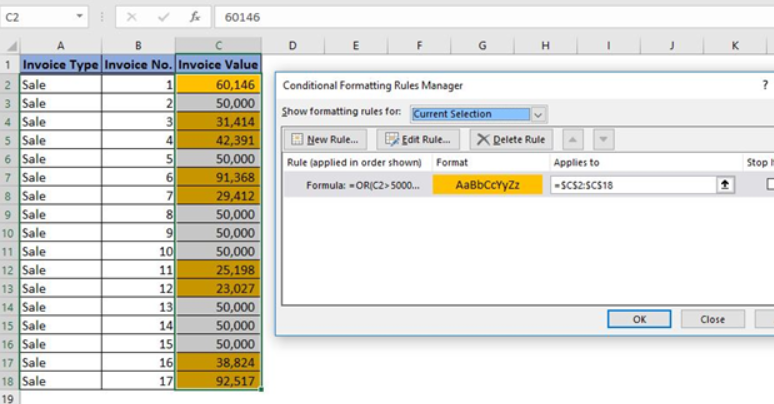Figure 9 – Rules Manager showing only one rule

## 2 Columns, 1 Rule, 2 Conditions

Up till now, we were applying 2 conditions on a single column but what if we wanted to apply 2 conditions on separate columns? Well, we will look at the solution to this problem now. As a start, let’s change our sales data to include purchase as well. (Figure 10)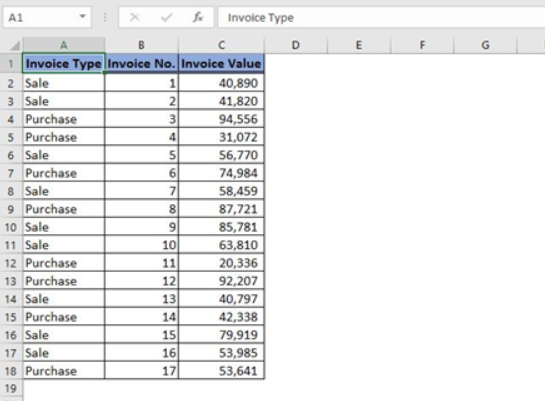Figure 10 – Data for purchase and sale

In the above data, we will highlight only those values that are greater the \$50,000 and pertain only to sales. To achieve this, we will do the following:

• Select the data range containing the invoice values.
• Go to the conditional formatting dialog box. “Home>Conditional Formatting>New rule”.
• Select “Use a formula to determine which cells to format”. The formula that we will apply, to select our invoices that have a value of greater than \$50,000 and pertain only to sales, is =AND(A2=“Sale”,C2>50000).
• Click “Format” to display the Format Cells dialog box, choose the format as per your liking. (Figure 11)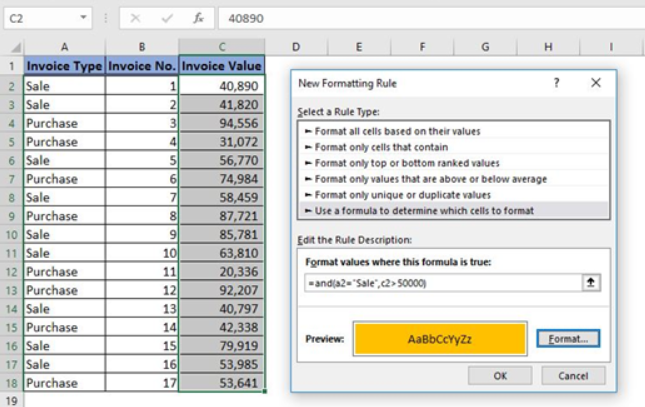Figure 11 – Application of 2 conditions on separate columns

In the above formula, we have used “AND” operator to test two conditions to identify sales invoices having a value greater than \$50,000. After application of formatting rules click “OK”. The final result will be highlighted sales invoices having a value greater than \$50,000. (Figure 12)Figure 12 – Final result of the application of testing two conditions on separate columns

## Instant Connection to an Expert through our Excelchat Service

Most of the time, the problem you will need to solve will be more complex than a simple application of a formula or function. If you want to save hours of research and frustration, try our live Excelchat service! Our Excel Experts are available 24/7 to answer any Excel question you may have. We guarantee a connection within 30 seconds and a customized solution within 20 minutes.

Are you still looking for help with Conditional Formatting? View our comprehensive round-up of Conditional Formatting tutorials here.

### Did this post not answer your question? Get a solution from connecting with the expert.Another blog reader asked this question today on Excelchat:
Solution examplesHi, I am trying to find an average of one column 'C', where another column = a particular value, or a third column = another particular value.
Solved by T. C. in 19 minsI need a formula where s36 = 1 if a10=s7 and c10=any value?
Solved by G. J. in 13 minsHello, I need help. (Excel) Assume that columns A, C and E in a worksheet contain the names of three adults who are interested in joining the (AARP), 50 years of age and over improve the quality of their lives. Columns B, D and F contain their respective ages in whole years in the adjacent cells. Which of the following formulas should be placed in cell G1 to verify that anyof these individuals are eligible to become full members, if they are at least 50 years old? =AND(B1>49, D1>49, F1>49) =OR(B1>=50, AND(D1>=50, F1>=50)) =OR(B1>=50, D1>=50, F1>=50) =AND(B1>=50, D1>=50, F1>=50
Solved by F. Q. in 20 minsI need a long If and AND statement. If a1 is between 17-21 AND a2 is greater than 42 and a3 is greater 53 and a4 is less than the time of 15:54 then they pass, if not they fail
Solved by E. S. in 54 minsWould like to know how use the filter in excel with AND instead of OR So filtering three columns with a 0 and 1 and asking that all three show up if at least one has a number 1
Solved by B. E. in 20 mins## Subscribe to Excelchat.coAnother blog reader asked this question today on Excelchat: